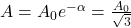## In damped harmonic oscillation, the amplitude of oscillation becomes one third after 2 second. If A0 is initial amplitude of oscillation, th

Question

In damped harmonic oscillation, the amplitude of oscillation becomes one third after 2 second. If A0 is initial amplitude of oscillation, then amplitude after 1 second from start will be

in progress 0
3 months 2021-08-15T07:41:04+00:00 1 Answers 6 views 0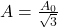Explanation:

Initial amplitude=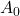We are given that

Amplitude after 2 s=A=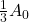We have to find the amplitude after 1 s.

We know that amplitude at any time t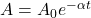Using the formula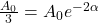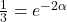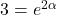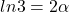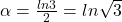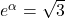When t=1 s